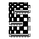622瀏覽
The Garch (General Autoregressive Conditional Heteroskedasticity) model is a non-linear time series model that uses past data to forecast future variance.

The Garch (1,1) formula is:

Garch = (gamma * Long Run Variance) + (alpha * Squared Lagged Returns) + (beta * Lagged Variance)

The gamma, alpha, and beta values are all weights used in the Garch calculations. According to RiskMetrics by JP Morgan, the optimal beta weight is 0.94, but this figure is highly disputed in the academic realm. The biggest problem academics and economists have with the 0.94 figure is that JP Morgan used monthly data to come to this number, meaning it does not take other time frames into account. Because of the disputed nature of what beta should be, this script will automatically calculate the beta weight for you in real time, taking into account the time frame you're using and realized variance, by using the Minimum Sum of Squared Errors Method.

The gamma and alpha weights are also calculated for you.

Even though the Garch formula provides today's projected variance, today's projected deviation is also calculated. This is done by taking the square root of Garch.

Additionally, if you want to project the variance or deviation for as many days forward as you want, you can.

In order to project the variance and deviation beyond just today, these equations are used:

Projected Variance = Long Run Variance + (alpha + beta)^Days Forward * (Garch - Long Run Variance)

Projected Deviation = sqrt(Projected Variance)

How to use this model:
1st. Decide the type of data you want: Projected Variance in % or Projected Deviation in %.
2nd. Decide how many days you want projected forward. If you input 0, you will get projections for today. If you input 1, you will get projections for tomorrow, and etc.

That's it. If you have any further questions, I left detailed comments in the code explaining each step, as best as I could.

Meanwhile, a few things that should be clarified from the previous version's description:

1. The (1,1) in GARCH (1,1) means that the model is using data from the most recent period to make projections.

2.In the previous description I said that JP Morgan advised using a Beta weight of 0.94 for all time frames. This is a mistake. Actually, they advised using 0.94 for daily data, and 0.97 for monthly data. Despite this, like I said in the original description, there is dispute over whether these values should be used, and their numbers don't take into account other time frames. This is why the SSE method is used in this script to calculate the optimal weights for you.

3.The Beta weight determines how closely the prediction will be to the previous period's variance.

4. The Alpha weight determines how volatility reacts to new information.

5. The Gamma weight determines the degree to which the Garch model's projections will revert to the mean, aka the Long Run Variance.

6. The (Alpha+Beta) component of the Projected Variance equation in the original description, determines the tendency of the projection to stick to the Long Run Variance. This is important to know because Alpha+Beta+Gamma must equal 1. This means that the higher (Alpha+Beta) is, the smaller Gamma will be, and vice versa. As stated above, Gamma determines the degree to which the projections will revert to the Long Run Variance. So the larger (Alpha+Beta) is the slower the rate of reversion will be; meanwhile the smaller (Alpha+Beta) is the quicker the rate of reversion will be.

For Example:
(the numbers presented below are solely for illustrative purposes)

Scenario A: Let's say you are projecting the variance of an asset over the next 5 days using this Garch model, where the Long Run Variance is 1%, (Alpha+Beta)=0.9, and Gamma=0.1. The projections for the next 5 days may look something like this: .

Scenario B: Let's say you are projecting the variance of an asset over the next 5 days using this Garch model, where the Long Run Variance is 1%, (Alpha+Beta)=0.7, and Gamma=0.3. The projections for the next 5 days may look something like this: .

In Scenario B Gamma is higher than in Scenario A, and because of this the projections for the next five days revert to the Long Run Variance at a much quicker rate than in Scenario A.

I hope this clarifies things better.

(the numbers presented below are solely for illustrative purposes)

Scenario A: Let's say you are projecting the variance of an asset over the next 5 days using this Garch model, where the Long Run Variance is 1%, (Alpha+Beta)=0.9, and Gamma=0.1. The projections for the next 5 days may look something like this: (Today: 1.6%, Day 1: 1.5%, Day 2: 1.4%, Day 3: 1.3%, Day 4: 1.2%, Day 5: 1.1%).

Scenario B: Let's say you are projecting the variance of an asset over the next 5 days using this Garch model, where the Long Run Variance is 1%, (Alpha+Beta)=0.7, and Gamma=0.3. The projections for the next 5 days may look something like this: (Today: 1.6%, Day 1: 1.45%, Day 2: 1.3%, Day 3: 1.15%, Day 4: 1.0%, Day 5: 1.0%).

In Scenario B Gamma is higher than in Scenario A, and because of this the projections for the next five days revert to the Long Run Variance at a much quicker rate than in Scenario A.

## 評論Great work! Hopefully one day we can see an autogarch fitter (for determining values other than (1,1)) in pine!